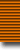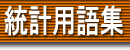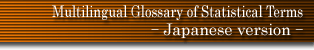### 用語検索

and or
検索単位 単語 フレーズ 完全一致 〜で始まる 〜を含む 〜で終わる 日本語 英語 ドイツ語 フランス語
 日本語 英語 ドイツ語 フランス語
 Sacks' theorem saddlepoint approximation same order bias estimators sample sample census sample design sample moment sample plan sample point sample reuse sample size sample space sample statistic sample survey sample unit sampling distribution sampling error sampling for attribute sampling fraction sampling inspection sampling interval sampling moment sampling on successive occasions sampling ratio sampling structure sampling survey sampling unit sampling variance sampling with replacement sandwich estimator Satterthwaite's approximation Satterthwaite's test saturated model saturation SB,SU distributions scale parameter scatter coefficient scatter diagram scatter plot scattergram scedastic curve scedasticity Schach's two sample tests schedule Scheffé's test scheme of hidden periodicity Schuster periodogram Schwarz's inequality score score test scree test screening design screening inspection S-curve seasonal fluctuation seasonal variation second limit theorem second order stationarity second order stationary secondary process secondary unit second-order analysis secretary problem sector chart secular trend segmented regression selected points method selection with arbitrary (variable) probability selection with equal probability selection with probability proportional to size self-avoiding random walks self-conjugate Latin square self-correlation coefficient self-renewing aggregate self-weighted sample self-weighting sample semi range semi-interquartile range semi-invariant semi-Latin square semi-logarithmic chart semi-logarithmic graph semi-Markov process semi-martingale semi-Normal distribution semi-range semi-stable law semi-stationary process Semivariogram sensitive information sensitivity and specificity sensitivity data sequential analysis sequential chi-square test sequential estimation sequential probability ratio test sequential selection sequential T test sequential test sequential tolerance region serial cluster serial correlation serial design serial sampling inspection schemes serial variation serially balanced sequence series series queues series seriation seriola Shannon-Wiener index shape parameter Shapiro-Wilk test Sheppard's corrections Sherman's test statistic Shewhart control chart shock and error model shock model Short distribution short term fluctuation shortest confidence intervals shorth shot noise Siegel-Tukey test sigmoid sigmoid curve sign test signed rank test significance significance level similar action similar regions similarity similarity index simple abnormal curve simple hypothesis simple lattice design simple random sampling simple sample simple structure simple table simplex algorithm simplex centroïd design simplex designs simplex method simulation model simulator simultaneous confidence intervals simultaneous discrimination intervals simultaneous equations model simultaneous estimation simultaneous tolerance intervals simultaneous variance ratio test single blind single factor theory single sampling single sampling plan single-linkage clustering single-tail test singly linked block design singular distribution singular value decomposition singular weighing design sinusoidal limit theorem six point assay size size and shape size of a region size of a test skew correlation skew distribution skew regression skewness skip free process Skitovich-Darmois theorem slippage test slope ratio assay Slutsky process Slutsky's theorem Slutsky-Yule effect Smirnov tests Smirnov-Birnbaum-Tingey distribution smooth regression analysis smooth test smoothing smoothing power SMR snap reading method Snedecor's F-distribution snowball sampling sojourn time space-time clustering spatial point process spatial systematic sample Spearman estimator Spearman two-factor theorem Spearman-Brown formula Spearman-Brown prophesy formula Spearman-Kärber estimator Spearman-Kärber method Spearman's ρ Spearman's footrule Spearman's rank correlation coefficient Spearman's rho species of abundance problem species of Latin square specific factor specific rate specification bias specification error specificity spectral average spectral decomposition spectral density spectral distribution function spectral function spectral weight function spectral window spectrum Spencer formula spent waiting time spherical distribution spherical mean direction spherical Normal distribution spherical standard error spherical variance spherical variance function spherically symmetric distributions sphering Spitzer's identity splicing spline estimate split half method split plot confounding split plot design split plot method split test method spread spreadsheet spurious correlation square contingency square lattice square root transformation squariance St Petersburg paradox stabilisation of variance stability test stable law stable Paretian distributions stable Pareto distributions stable process (distribution) stable state Stacy's distribution staircase design staircase distribution staircase method standard deviate standard deviation standard equation standard error standard error of estimate standard Latin square standard measure standard Normal variate standard population standard score standardised deviate standardised mortality ratio standardised regression coefficients standardised variate stationary distribution stationary population stationary process statistic statistical decision function statistical hypothesis statistical population statistical tolerance interval statistical tolerance limit statistical tolerance region statistical universe statistically equivalent block statistics Steiner's triple systems Stein's paradox Stein's two sample procedure stem and leaf display stem and leaf plot step down procedure Stephan's iterative process stepwise regression STER distribution stereogram stereology s-test Stevens-Craig distribution Stirling distribution stochastic stochastic abundance models stochastic approximation procedure stochastic comparison of tests stochastic continuity stochastic convergence stochastic dependence stochastic differentiability stochastic disturbance stochastic integrability stochastic kernel stochastic matrix stochastic model stochastic process stochastic programming stochastic transitivity stochastic variable stochastically larger or smaller stopping rule strata chart strategy stratification stratification after selection stratified sample stratum strength of a test stress strictly dominated strictly stationary process strong completeness strong law of large numbers strongly consistent estimator strongly distribution free structural equation structural parameters structure studentisation studentised maximum absolute deviate studentised range Student's distribution Student's hypothesis Student's t-distribution Student's test Sturges' rule subexponential distribution sub-group confounded subjective Bayesian inference subjective probability submartingale subnormal dispersion sub-Poisson distribution Subrahmaniam's generalised contagious distribution subsample subsampling sub-series substitute F-ratio substitute t-ratio substitution successive difference statistic sufficiency Sukhatme d-statistic Sukhatme's test sum of squares about the mean superefficiency superfluous variable super-martingale supernormal dispersion super-Poisson distribution superposed process superposed variation supersaturated design supplementary data supplementary information supplemented balance support surprise index survey survey design survival analysis survival function survivor function swindle switchback design symmetric circular distribution symmetric sampling symmetric stable law symmetrical distribution symmetrical factorial design symmetrical test symmetrical unequal block arrangement symmetry sympathy effect systematic systematic design systematic error systematic sample systematic square systematic statistic systematic variation